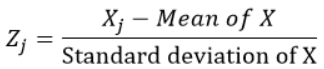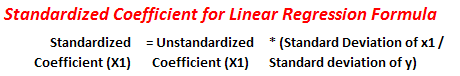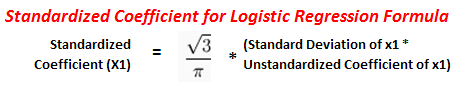CHIRAG GOYAL — Published On March 21, 2021 and Last Modified On February 24th, 2023

## Introduction

Some time back, I was making the predictive model using Linear Regression, and I found a variable whose unstandardized coefficient of regression (beta coefficients or estimate) was close to zero. Still, after some analysis, I found it statistically significant (means p-value <0.05). We know that if a variable is significant for a particular model, its coefficient value is significant and different from zero if the effect size is also significant. So, the question that arises is, “Why is the coefficient value close to zero, but that variable is significant for our predictive model?”.

The answer to this question lies in the difference between the standardized and unstandardized coefficients of regression. So, in this article, we will see the basic concepts behind these coefficients and how they differ from each other, with their advantages and disadvantages.

Learning Objectives

• Understand what standardized and unstandardized regression coefficients are.
• Find out the use cases of standardized regression coefficients.
• Learn to calculate regression coefficients.

.

This article was published as a part of the Data Science Blogathon.

## Unstandardized Regression Coefficients

#### What are unstandardized regression coefficients?

Unstandardized coefficients are those that the linear regression model produces after its training using the independent variables, which are measured in their original scales, i.e., in the same units in which we are taken the dataset from the source to train the model.

An unstandardized coefficient should not be used to drop or rank predictors (aka independent variables) as it does not eliminate the unit of measurement. For Example, let’s take a hypothetical multiple regression example where we want to predict the income(in rupees) of a person based on their age (in years), height(in cm), and weight(in kg). So, here inputs for our regression analysis are age, height, and weight, and the output(response variable) is income. Then,

Income(rupees)=a0+a1*age(years)+a2*height(cm)+a3*weight(kg)+e                (eqn-1)

#### How to Interpret Unstandardized Regression Coefficients?

These are used to interpret the effect of each independent variable on the outcome(response/output). Their interpretation is straightforward and intuitive. All other variables held constant; a 1 unit change in Xi(predictors) implies there is an average change of ai units in Y(outcome).

In the above example of multiple linear regression, if a1=0.3, a2=0.2, and a3=0.4 (and assume all are statistically significant), then we interpret these coefficients as follows:

Getting 1 year older is associated with an increase of 0.3 in income, assuming other variables are constant (which means there is no change in height and weight). Similarly, we can interpret the coefficient for other independent variables as well.

It represents the amount by which dependent variable changes if we change independent variable by one unit keeping other independent variables constant.

#### Limitations of Unstandardized Regression Coefficients

Unstandardized coefficients are great for interpreting the relationship between an independent variable X and an outcome Y. However, they are not useful for comparing the effect of an independent variable with another one in the model.

For Example, which variable has a larger impact on Income? Age, Height, or weight?
We can try to answer this question by looking at equation-1 and again assume that a1=0.3, a2=0.2, and a3=0.4, we conclude that :

“An increase of 20 cm in height has the same effect on the weight increases 10 times” Still, this does not answer the question of which variable affects Income more.

Specifically, the statement that “the effect of the increase of weight by 10 times = the effect of the increase in the height by 20 cm” is meaningless without specifying how hard it is to increase height by 20 cm, specifically for someone who’s not familiar with this scale.

So, at last, we conclude that a direct comparison of the regression coefficients for any of the pair of independent variables is not making sense or is not useful as these independent variables are on different scales (age in years, weight in kg, and height in cm).

It turns out that the effects of these variables can be compared by using the standardized version of their coefficients. And that’s what we’re going to discuss next.

## Standardized Regression Coefficients

#### What Are Standardized Regression Coefficients?

The concept of standardization or standard coefficients is used in data science when independent variables or predictor variables for a particular model are expressed in different units. For Example, let’s say we have three independent features of a woman: height, age, and weight. Her height is in inches, her weight in kilograms, and her age in years. If we want to rank these predictors based on the unstandardized coefficient (which directly comes when we train a regression model), it would not be a fair comparison since the units for all the predictors are different.

The standardised coefficients of regression are obtained by training(or running) a linear regression model on the standardized form of the variables.

The standardized variables are calculated by subtracting the mean and dividing by the standard deviation for each observation, i.e., calculating the Z-score. It would make mean 0 and standard deviation 1. For this, they also need to follow the normal distribution. Then, they don’t represent their original scales since they have no unit.

For each observation “j” of the variable X, we calculate the z-score using the formula:Which variables do we have to standardize for finding the standardized regression coefficients, i.e., both predictor and response or either one of them?

Yes, we standardize both the dependent(response) and the independent(predictor) variables before running the linear regression model (as this is the widely accepted practice when we want to find the standardized form of the variables).

#### How to Interpret the Standardized Regression Coefficients?

The interpretation of standardized regression coefficients is non-intuitive compared to their unstandardized versions: For example, a 1 standard deviation unit increase in X will result in β standard deviation units increase in y.

A change of 1 standard deviation in X is associated with a change of β standard deviations of Y.

Note:

– If there is a categorical variable in place of a numerical variable in our analysis, then its standardized coefficient cannot be interpreted as it does not make sense to change X by 1 standard deviation. In general, this is not a problem for our model since these coefficients are not meant to be interpreted individually but to be compared to one another in order to get a sense of the importance of each variable in the linear regression model.

The standardized coefficient is measured in units of standard deviation. A beta value of 2.25 indicates that of one standard deviation increase in the independent variable results in a 2.25 standard deviations increase in the dependent variable.

#### What Is the Real Use of Standardized Coefficients?

They are mainly used to rank predictors (or independent or explanatory variables) as they eliminate the units of measurement of independent and dependent variables). We can rank independent variables with an absolute value of standardized coefficients. The most important variable will have the maximum absolute value of the standardized coefficient.

For example:

Y = β0 + β1 X1 + β2 X2 + ε

If the standardized coefficients β1 = 0.5 and β2 = 1, we can conclude that:

X2 is twice as important as X1 in predicting Y, assuming that both X1 and X2 follow roughly the same distribution and their standard deviations are not that different.

#### Limitations of Standardized Regression Coefficients

The standardized coefficients are misleading if the variables in the model have different standard deviations means all variables are having different distributions.

Take a look at the following linear regression equation:

Income(\$) = β0 + β1 Age(years) + β2 Experience(years) + ε

Because our independent variables, Age and Experience, are on the same scale (years) and if it is reasonable to assume that their standard deviations differ a lot, then in this case:

• Their unstandardized coefficients should be used to compare their importance/influence in the model.
• Standardized these variables would, in fact, cause them to be on a different scale (different standard deviations or follows different distribution)

## Calculation of Standardized Coefficients

#### For Linear Regression

(Another approach as we see one approach in the above part of the article)

The standardized coefficient is found by multiplying the unstandardized coefficient by the ratio of the independent and dependent variable standard deviations.#### For Logistic RegressionWe calculate them using various software like spss, sas, R, and Python.

## Conclusion

This article covered some basic but necessary concepts that come in handy while working on real-life projects in Machine Learning and Artificial Intelligence. Towards the end of this article, we’ve looked into the Mathematics behind these concepts and also learned to calculate regression coefficients. Not that both standardized and unstandardized coefficients have their own separate use cases and must therefore be chosen based on the data set and need.

Key Takeaways

• Unstandardized coefficients are found by training a linear regression model using the independent variables, measured in the same units as the source or raw data set.
• You can find the standardized coefficients of regression by training a linear regression model on the standardized form of the variables.
• Standardized variables are calculated by subtracting the mean and dividing the answer by the standard deviation for each observation.

#### Q1. Should I use standardized or unstandardized coefficients in regression?

A. Both of them have their use cases. So, it’s better to calculate both and interpret them accordingly.

#### Q2. How do I find the standardized coefficient of a variable?

A. standardized coefficient is found by multiplying the unstandardized coefficient by the ratio of the independent and dependent variable standard deviations. It can be interpreted as 1 standard deviation change in feature results in coefficient times standard deviation change in the y variable.

#### Q3. What is the difference between B and β in simple linear regression?

A. B corresponds to the unstandardized coefficients, while β corresponds to the standardized coefficients.